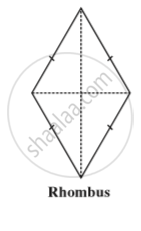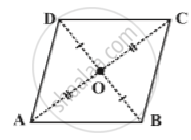Share

# Some Special Parallelograms - Concept of Rhombus

#### notes

Rhombus is also a parallelogram.These are ,
1) The opposite angles of a rhombus are equal.
2) The opposite sides of a rhombus are parallel to each other.
3) The adjacent angles of a rhombus are supplementary.
4) The diagonals of a rhombus bisect each other.
There are exceptional properties of rhombus are
1) All the sides of a rhombus are equal.
2) The diagonals of a rhombus bisect each other at right angles.

Property: The diagonals of a rhombus are perpendicular bisectors of one another.ABCD is a rhombus. Therefore it is a parallelogram too. Since diagonals bisect each other,
OA = OC  and  OB = OD.
We have to show that m∠AOD = m∠COD = 90°
It can be seen that by SSS congruency criterion
∆ AOD ≅ ∆ COD
Therefore, m ∠AOD = m ∠COD
Since ∠AOD and ∠COD are a linear pair,
m ∠AOD = m ∠COD = 90°

### Shaalaa.com

Property: The diagonals of a rhombus are perpendicular bisectors of one another [00:04:04]
S
0%

S